Ioelovich, M., and Leykin, A. (2011). "Study of sorption properties of cellulose and its derivatives , " BioRes. 6(1), 178-195.

#### Abstract

The sorption of vapors by cellulose samples and by some cellulose derivatives was studied at 25 oC. To describe sorption isotherms, a thermodynamic equation was proposed: A=Ao/[1-(RT/g)lnφ], where Ao is maximal sorption value, φ is relative pressure of vapors, and g is specific thermodynamic potential. Depending on the g -value, this equation can describe isotherms of various shapes that occur for cellulose and its derivatives. Application of the equation makes it possible to calculate such structural characteristics of the polymers as accessible specific surface and crystallinity, as well as the substitution degree of cellulose derivatives. Moreover, amounts of monomolecular and multimolecular fractions of the sorbate can be determined.

STUDY OF SORPTION PROPERTIES OF CELLULOSE AND ITS DERIVATIVES

Michael Ioelovich* and Alex Leykin

The sorption of vapors by cellulose samples and by some cellulose derivatives was studied at 25 oC. To describe sorption isotherms, a thermodynamic equation was proposed: A=Ao/[1-(RT/g)lnφ], where Ao is maximal sorption value, φ is relative pressure of vapors, and g is specific thermodynamic potential. Depending on the g-value, this equation can describe isotherms of various shapes that occur for cellulose and its derivatives. Application of the equation makes it possible to calculate such structural characteristics of the polymers as accessible specific surface and crystallinity, as well as the substitution degree of cellulose derivatives. Moreover, amounts of monomolecular and multimolecular fractions of the sorbate can be determined.

Keywords: Cellulose; Derivatives of Cellulose; Vapors; Sorption; Isotherms; Calculations

Contact information: Polymate Ltd, P.O.Box 73, Migdal HaEmek 23100, Israel;

*Corresponding author: bd895892@zahav.net.il

INTRODUCTION

Sorption of gases and vapors is widely used for investigation and characterization of microstructure of various polymers such as cellulose, as well as its derivatives, e.g. cellulose ethers and esters. For testing of the specific surface area and porous structure of hydrophilic cellulose materials, vapors of relatively inert non- and low-polar organic liquids have been chosen as sorbates (Dollimore et al. 1973; Chirkova et al. 2007), while for the characterization porous structure of hydrophobic cellulose derivatives, the sorption of water vapor can be applied (Barnes et al. 1977; Gocho et al. 2000). Water vapor is the main sorbate used for study of the sorption properties and structure of various cellulose materials such as cellulose powders, films and fibers of regenerated cellulose, textile materials, pulp, paper, cardboard and some other materials (Blair et al. 1990; Hill et al. 2009; Jeffries 1960; Kawai 1959; Rowen et al. 1947; Urquhart 1959). Sorption of solvent vapors, e.g. sorption of water vapors by water-soluble cellulose ethers (Baumgartner et al. 2002) or sorption acetone vapors by cellulose esters (Mandelkern et al 1951), is also used to study the interaction between active sorbates and cellulose derivatives.

Sorption of vapors is usually described through isotherms having various shapes that express the relative amount of sorbate in the sorbent (A, g/g) as a function of relative vapor pressure (φ=P/Po) at a constant temperature (Gregg and Sing 1982). To calculate sorption parameters and structural characteristics of sorbents, the obtained isotherms should be linearized by the appropriate equations corresponding to the assumed sorption mechanism.

Isotherms of type I exhibit a saturation plateau due to adsorption of an inert sorbate on the surface of pores. In this case the Langmuir equation is used for linearization of the isotherms and for calculations of monolayer adsorption value (Am) and specific surface area (Ssp) of the sorbent (Sohn and Kim 2005).

A widely occurring case involves multilayer adsorption of inert sorbates onto the surface of mesoporous sorbents. Adsorption isotherms of this type are sigmoidal and related to type II or IV. The linearization of such isotherms is usually performed by means of the BET equation in order to calculate values of Am and Ssp, as well as an energetic constant (C) (Allen 1997; Brunauer et al. 1938; Gregg and Sing 1982). If φ is low and the C-constant is high, the BET equation turns into the Langmuir equation. Examinations showed that the BET equation provides linearization of the experimental isothems in a very limited φ-range, usually from 0.05 to 0.35.

Sorption isotherms of active polar vapors (methanol, water, ammonia, etc.) by cellulosic materials also have sigmoidal shape, similar to multilayer adsorption isotherms (Bariska et al. 1975; Chirkova at al. 2007; Jeffries 1960). However, the BET equation cannot be used for calculation of sorption characteristics, because sorption mechanism isn’t adsorption of the sorbate on the surface of pores, but absorption of the vapor molecules into non-crystalline (amorphous) domains of the polymer. This process is accompanied by swelling, plasticization, and other structural changes of the cellulose sorbent (Papkov and Fainberg 1976). High values of “imaginary” surface area of cellulose samples obtained by sorption of methanol vapors (Ssp = 160 m2/g: Chirkova et al. 2007), water vapors (Ssp = 245 m2/g: Chirkova et al. 2007), and vapors of ammonia (Ssp = 500 m2/g: Bariska et al. 1975) do not reflect a real surface area. In reality, it is a result of interaction between active molecules and polar groups of individual glucopyranose units inside the amorphous fractions of cellulose (Zografia et al. 1984).

Water absorption into amorphous domains of various cellulose samples including microcrystalline cellulose takes place in a manner similar to the absorption of water molecules by amorphous starches (Zografia et al. 1984). The absorption mechanism is confirmed by direct correlation between amount of the sorbed water and the content of non-crystalline (amorphous) domains of cellulose (Ioelovich et al. 1983 and 2009; Jeffries 1960; Hermans 1949; Mihranyan et al. 2004; Papkov and Fainberg 1976).

Special equations, e.g. equations of Sakurada-Ninishina and De Boer-Zwicker, based on absorption theory have been used to explain the shapes of isotherms of water vapor sorption by cellulose (Papkov and Fainberg 1976). To describe exponential sorption process of solvent vapors by soluble polymers having isotherms of III or V types, the Flory-Huggins type equation is required (Tager 1978).

Thus, several different equations have been applied to describe sorption properties of various vapors by cellulose and its derivatives. However, these equations usually have correlated poorly with experimental results, a lack of fit that hinders determination of sorption parameters and structural characteristics of the polymer sorbents. Additional problems are due to the complicated sorption of vapors by the cellulose and its ethers or esters, since in many cases various sorption processes such as surface adsorption, volume absorption, capillary condensation, unrestricted swelling and some others, can take place simultaneously. The detailed investigation of the vapor sorption requires finding an approach for isolation of the individual sorbate fractions related to different sorption mechanisms.

In this paper for calculation of main types of isotherms that occur for cellulose and its derivatives, a simple thermodynamic equation is proposed. Application of this equation for determination of monomolecular and multimolecular fractions of sorbates and calculation of structural characteristics of the polymers is discussed.

EXPERIMENTAL

Materials

The following cellulose samples were investigated:

• Microcrystalline cellulose (MCC) Avicel PH-301 of FMC BioPolymer Co.
• Pure chemical grade cotton cellulose (COC) of Hercules Co.
• Mercerized cotton cellulose (COCM) prepared by treatment with 20% NaOH and following washing and drying
• Bleached high-pure sulfite spruce pulp (SFI) of Weyerhaeuser Co.
• Regenerated viscose fibers (VF) of Rayonier Inc.
• Regenerated cellophane film (CF) of Celulozon Co.
• Porous cellophane film (CFP) obtaining from the initial CF by its swelling in water and exchange with absolute ethanol and then with pentane and evaporation of pentane at 60 oC up to constant weight of the sample
• Sodium carboxymethylcellulose (CMC) of Sigma-Aldrich having degree of substitution degree DS=0.6.
• Hydroxyethylcellullose (HEC) “Natrosol” of Hercules, DS=2.4.
• Methylcellulose (MC) “Methocel” of Dow Chemicals, DS=1.8.

X-Ray Diffraction

A Rigaku-Ultima Plus diffractometer (CuK – radiation, =0.15418 nm) was used for X-ray investigations. Diffractograms were recorded in the φ=2 angle range from 5 to 80. After recording of the diffractograms, the background was separated, and selected X-ray patterns were corrected and normalized. Then diffraction intensities from crystalline and non-crystalline regions were separated by a computerized method. The degree of crystallinity (X) was calculated according to equation,

X = ∫ Jc dφ / ∫ Jo dφ (1)

where Jc and Jo are the corrected and normalized diffraction intensities for crystalline regions and sample, respectively.

The content of amorphous domains (Y) in a cellulose sample, as determined by the X-ray method, was calculated as:

Y = 1 – X (2)

A detailed description of the X-ray diffraction method can be found in the papers of Ioelovich et al. (1992, 1994, and 2009).

Testing of Specific Surface

Specific surface (SN2) of the samples was measured at -196 oC by nitrogen adsorption using Sorptometer QBET-NV1A of Porous Material Inc.

Characteristics of Samples

Some characteristics of the investigated samples are shown in Table 1.

Table 1. Characteristics of celluloses and cellulose derivatives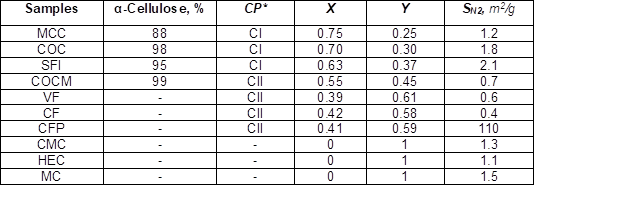*Note: CP signifies a Crystalline Polymorph. CI and CII are crystalline polymorphs of cellulose having different parameters of elementary crystalline cell (Bikales and Segal 1971). CI polymorph is transformed into CII polymorph during mercerization of native cellulose or regeneration from cellulose solutions.

As follows from Table 1, the investigated samples exhibited various degrees of crystallinity (from 0 to 0.75), content of amorphous domains (from 0.25 to 1), and specific surface area (SN2) measured by nitrogen adsorption (from 0.4 to 110 m2/g). Dried samples of cellulose and its derivatives were characterized by low values of SN2: 0.4-2.1 m2/g. This is in agreement with results of other researchers (Chirkova et al. 2007; Papkov and Fainberg 1976; Tager 1978; Zografia et al. 1984). Only by use of special methods based on exchanging of water from swollen samples with a volatile low-polar organic liquid and evaporation at decreased temperatures is it possible to obtain samples with developed specific surface, e.g. CFP-sample having SN2=110 m2/g. It can be mentioned also that the method of Weatherwax and Caulfield (1971) allows one to achieve a high specific surface (about 200 m2/g) of cellulose samples via exchange of water from swollen cellulose with ethanol, then exchange of ethanol with liquid carbon dioxide, which is evaporated above critical temperature.

Sorption of Vapor

The sorption experiments were carried out at 25 oC on a vacuum Mac-Ben apparatus having helical spring quartz scales. Prior to starting of the experiments the samples were dried at 105 oC up to constant weight and additionally degassed in the sorption device.

RESULTS AND DISCUSSION

The proposed sorption equation is based on the fundamental equation of Gibbs-Duhem for the thermodynamic equilibrium in a binary system:

N1 Δμ1 + N2 Δμ= S ΔT + V ΔP, (3)

where N1 and N2 are parts of the components in the binary system; Δμ1 and Δμ2 are specific thermodynamic potentials of the components; S is enthalpy; V is volume; T is temperature, and P is pressure.

At the isothermal conditions, ΔT=0 and

N1 Δμ1 + N2 Δμ= V Δ(4)

A solid polymer sorbent can have lots of sorption centres of various nature. Both adsorption onto surfaces and absorption into bulk material need to be considered. For the process of physical adsorption of vapors by the porous sorbent these centers are links or functional groups of the macromolecules located on surface of the pores having excess of the free energy, while in the case of absorption such centres can be links or groups inside the volume of the polymer sorbent. When sorbate vapors are injected in a closed reservoir containing polymer sorbent, a dynamic process between the coupling of molecules to the sorption centres and the reverse, i.e. detachment of these molecules, are started and the process continues until establishment of an equilibrium state. As a result, one part of the sorbent centres Θ becomes occupied by molecules of the sorbate, while another part of the centres 1Θ detaches of the molecules and becomes vacant. At the equilibrium state ΔP=0; and then use of the isothermal Gibbs-Duhem equation (4) for the occupied and vacant centers gives:

Θ Δμ + (1– Θ) g 0 (5)

Since Δμ=RT lnφ is the specific potential of the occupied part of the sorbent centers at a relative pressure of the vapors φ=P/Po, then:

Θ = 1[1-(RT/glnφ] (6)

Taking into consideration that Θ=A/Ao, the sought sorption function A=F(φ) will be the following:

A=Ao[1(RT/g) lnφ] (7)

or in the linear form,

1/A = 1/Ao – (K/Ao) lnφ (8)

where A is the sorption value at the certain relative pressure of the vapors φ, and Ao is the maximal sorption value in the presence of saturated vapors, φ=1; g is average specific potential of the vacancy forming via detaching of one molecule of the sorbate from one sorption centre that for the given sorbent-sorbate system is postulated as constant, and K=RT/g.

The proposed equations (6), (7), and (8) have the following features:

Feature 1.

According to Eq. (8), extrapolation of the linear plot 1/A=F(lnφ) to lnφ = 0 gives the parameter g and maximal sorption value Ao:

Ao=1/B and g=-RT/(n Ao) (9)

where B is intercept and n is slope coefficient of the plot 1/A =F(lnφ)

Feature 2.

Since the proposed equations (6), (7), and (8) are based on thermodynamic laws, they can be fit for various sorption processes, both for adsorption and absorption. The statement = constant is true under the condition that not more than one sorbate molecule is joined with one sorption centre, i.e. when the maximal Θ-value (Θo) equals one. This condition can be fulfilled in the case of monomolecular (monolayer) surface adsorption, limited monomolecular surface absorption, or monomolecular absorption inside the volume of the polymer sorbent.

Feature 3.

Calculated isotherms of monomolecular sorption can have various shapes and types depending on the g-value. If g-value is high (tens or hundreds kJ/mole), then an isotherm will have the saturation plateau, i.e. Γ-shape. At a medium g-value (some unities kJ/mole) a sigmoidal or S-shape isotherm can be obtained, while a very low g-value leads to an exponential isotherm (see Fig. 1).

Feature 4.

If the monomolecular sorption is complicated by multimolecular sorption processes (i.e. when Θo >1), then the application of the eq. (7) makes it possible to isolate individual fractions of the isotherm having different sorption mechanisms. After that the Aovalue is determined, and the accessible specific surface of the sorbent can be calculated,

S= D s Ao (10)

where is a dimensionality coefficient, and s is the cross-section area of the sorbed molecules (Allen 1997; Gregg and Sing 1982; Tager 1978).

In the case of inert non-swelling sorbates Sa is equvalent to the specific surface of pores in the sorbent.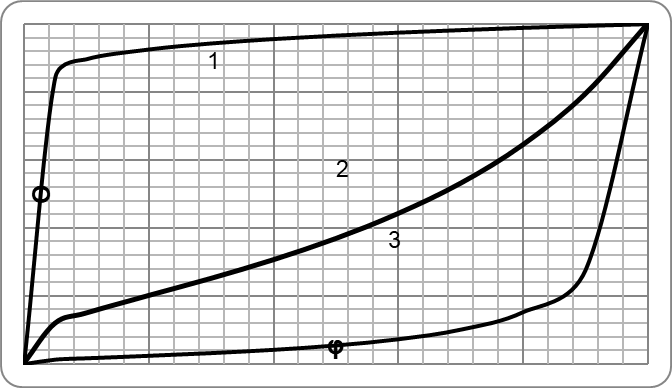Fig. 1. Theoretic sorption isotherms calculated by eq. (9) at g=40 (1), g=1 (2) and g=0.1 kJ per mole of a sorbate (3)

The following examples illustrate feature 4:

4.1. Sorption of vapors of n-hexane by cellulose samples

The sorption isotherms of the vapors by cotton cellulose (COC) and viscose fibers (VF) are similar to isotherms of type I having the saturation plateau (Fig. 2). Despite higher crystallinity, COC has some increased sorption than the less crystalline VF. This provides evidence that just surface adsorption occurs in the cellulose-hexane system.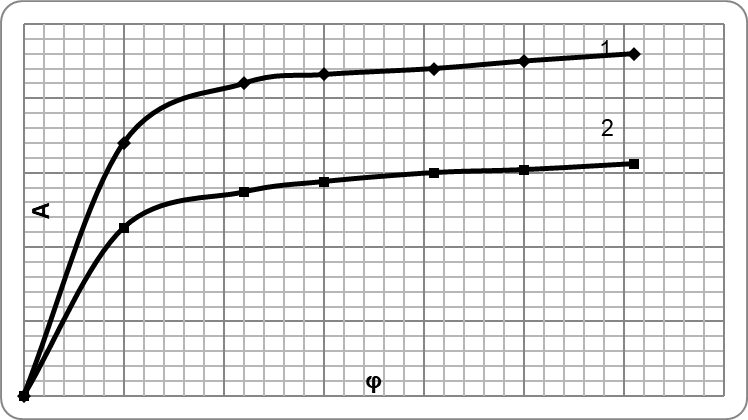Fig. 2. Calculated (line) and experimental (points) isotherms of hexane vapors sorption by cotton cellulose (1) and viscose fibers (2)

The isotherms were linearized by means of eq. (8) (see Fig. 3), which makes it possible to determine the sorption parameters Aog and K, and to calculate surface areas of the sorbents (Table 2), as well as the isotherms (Fig. 2). The increased parameter g =11.5 ± 0.5 kJ/mole for the cellulose-hexane system determines the Γ-shape of the isotherms.

Table 2. Sorption Parameters for the System Cellulose-Hexane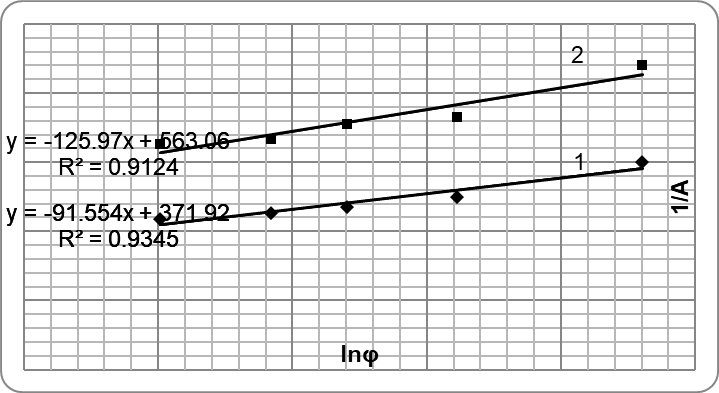Fig. 3. Linearized sorption isotherms of hexane vapors by cotton cellulose (1) and viscose fibers (2)

The results show that the surface area of cotton cellulose is higher than that of viscose fibers (Table 2). Moreover, sorption of hexane vapors gives higher specific surface area than sorption of nitrogen (Table 1). This is explained by a more developed porosity of the sorbent at the room temperature than at temperature of liquid nitrogen (Tager 1978).

4.2. Sorption of vapors of n-propanol by cellulose samples

The sorption process for n-propanol is characterized by sigmoidal isotherms and sorption hysteresis (Fig. 4). Cotton cellulose sorbs some more sorbate vapors than viscose fibers. After linearization of the isotherms in the φ-range 0.1 to 0.7 by means of Eq. (11), the sorption parameters Ao, gK and Sa , as well as isotherms were calculated (Table 3, Fig. 5). The sigmoidal form of the isotherms implies a medium value of the parameter g =1 ± 0.1 kJ/mole for the cellulose-propanol system.

Table 3. Sorption Parameters for the System Cellulose – Propanol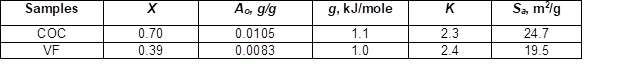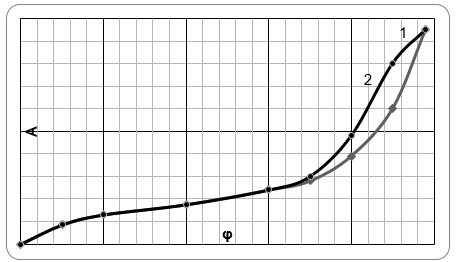Fig. 4. Sorption (1) and desorption (2) isotherms of propanol vapors by cotton cellulose

Increased areas of the accessible specific surface reflect access of polar sorbate molecules into the structure of the cellulose sorbents. Comparison of S– values shows that the accessibility of cotton cellulose to propanol is higher than that of viscose fibers. Probably, along with monomolecular adsorption, some limited monomolecular absorp-tion of the polar sorbate by surface layers of cellulose takes place. The isotherms related to this process were calculated by the Eq. (7). If φ <0.7, then the calculated adsorption or desorption isotherms are equal to the experimental data. However, at φ >0.7 the experimental isotherms deviate from the calculated values due to the starting of multimolecular sorption processes such as multilayer adsorption and capillary condensation, causing sorption hysteresis. Therefore, the experimental isotherms were separated into two individual fractions, one representing limited monomolecular surface sorption and the other involving additional multimolecular sorption (Fig. 5). The average radius of mesopores estimated by Kelvin’s equation for dry cellulose samples was in the range from 2 to 6 nm.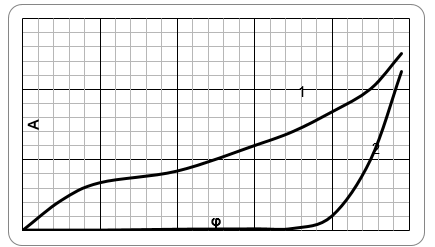Fig. 5. Monomolecular surface sorption (1) and multimolecular sorption (2) of propanol vapors by COC

Feature 5.

Application of the Eq. (7) to the sorption of active swelling sorbates by cellulose and its derivatives permits calculation of the crystallinity degree of the polymers. On the basis of this equation a substitution degree of the derivatives can be also estimated. Moreover, the monomolecular sorption can be separated from multimolecular sorption processes, e.g. capillary condensation, multimolecular absorption due to unrestricted swelling, etc.

Feature 5 can be illustrated by the following examples:

5.1. Sorption of water vapors by cellulose samples

Despite the sigmoidal form of the sorption isotherms (Fig. 6), the sorption mechanisms do not involve multilayer surface adsorption, but absorption of the water molecules into amorphous domains of cellulose (Ioelovich et al. 1983 and 2009; Jeffries 1960; Hermans 1949; Mihranyan et al. 2004; Papkov and Fainberg 1976). Therefore, with increasing content of amorphous domains in the samples the sorption value rises (Fig. 6).

Using the additive functions of Van Krevelen for sorption of water molecules by various groups of amorphous polymers (Van Krevelen 1972), the absorption values of water (Aam, g/g) into amorphous domains of cellulose (ADC) at various φ-ranges were calculated. After linearization of the isotherm (Fig. 7) the following absorption parameters were obtained: Aam,o = 0.5 g/g (at φ=1), = 0.95 kJ/mole, and K=2.61 (Table 4). This makes it possible to calculate the general absorption isotherm of the vapors by amorphous domains of cellulose (Fig. 6) by means of Eq. (7). If use the desorption isotherm of ADC, then the parameters of Eq. (7) will be: Aam,o = 0.5 g/g (at φ=1), = 1.1 kJ/mole, and K=2.17.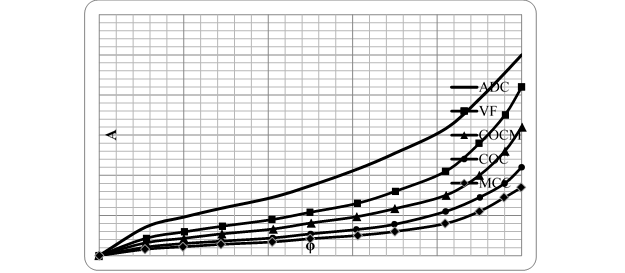Fig. 6. Isotherms of water vapors absorption by various cellulose samples and general absorption isotherm of amorphous domains of cellulose (ADC)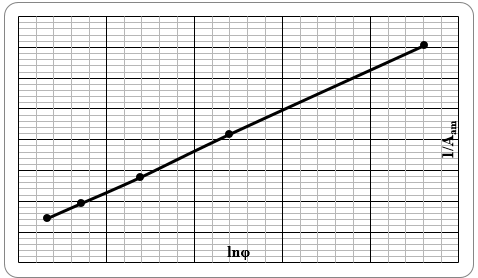Fig. 7. Linearized isotherm of water vapors absorption by amorphous domains of cellulose

Taking in consideration that the maximal absorption value for crystalline cellulose sample A= Aam,o(1-X)where X is the crystallinity degree, the final equation (11) for calculation of absorption isotherms of celluloses with various crystallinity or part of amorphous domains (Y) was derived:

A(g/g)0.5(1-X)/(1-2.61lnφ) = 0.5Y/(12.61lnφ) (11)

Since glucopyranose links of cellulose contain five polar groups (three OH- and two -O- groups), the maximal absorption value of amorphous domains Aam,o= 0.5 g/g at φ=1 corresponds to sorption of less than one water molecule by each polar group at 25 oC, and therefore it is really monomolecular absorption.

Table 4. Parameters of Water Vapor Sorption by Amorphous Domains of Cellulose (ADC) and Amorphous Derivatives Having Various Degrees of Substitution (DS)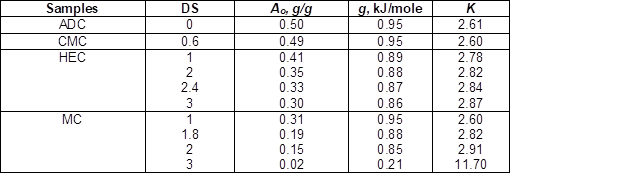Table 5. Maximal Contents of Absorbed (Ao) and Capillary Condensed (Ac) Water at φ =1 in Cellulose Samples having Various Structural Characteristics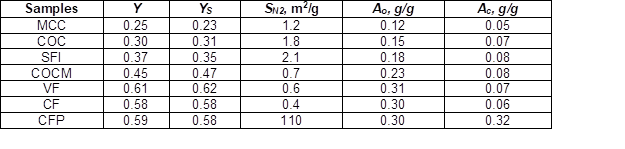By means of eq. (11) also structural characteristics of cellulose, XS and YS, can be determined from sorption experiments. It is convenient for this purpose to use the sorption value A0.7 at the relative pressure of vapors φ=0.7. Then:

XS = 1- 3.85 A0.7 (12)

and Y3.85 A0.7 (13)

The values of XS or YS calculated from results of water vapors sorption are correlated with these structural characteristics obtained by the X-ray method (Table 5).

At increased φ >0.85 the experimental isotherms deviate from the calculated due to starting of capillary condensation (Papkov and Fainberg 1976). Application of Eq. (11) permits separation of the absorbed and capillary condensed water (Table 5). Use of the BET equation for isotherms of water vapor sorption by cellophane films gives an “imaginary” specific surface area of 250 m2/g, which is higher than the real areas of specific surface (SN2) both for the low (SN2=0.4 m2/g) and highly porous (SN2 = 110 m2/g) cellophane samples. Therefore, despite a significant difference in SN2 values, these samples have the same content of water absorbed at φ < 0.85. However, at φ >0.85 the more porous sample is characterized by higher content of capillary condensed water (Fig. 8).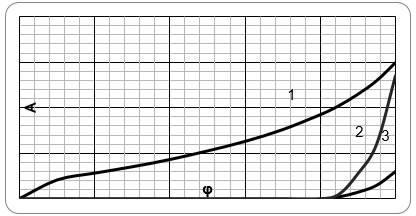Fig. 8. Adsorbed (1) and capillary condensed water for samples of highly porous CFP (2) and low porous CF (3)

The average radius of mesopores calculated by Kelvin’s equation for wet cellulose samples was in the range from 6 to 10 nm. Judging from content of capillary condensed water, volume of nano-pores in wet cellulose samples usually doesn’t exceed 0.08 cm3/g. Only for special prepared porous CFP-sample volume of nano-pores in the wet state is higher, about 0.3 cm3/g.

5.2. Sorption of water vapors by carboxymethylcellulose (CMC)

Due to a low degree of substitution (DS=0.6) and amorphous structure, the water vapors isotherm for CMC is similar to the isotherm of amorphous domains of cellulose over a wide φ-range (Fig. 9). Only at φ>0.85, an exponential increasing of water amounts in the sample is observed, probably due to unrestricted swelling, which continues up to forming a solution of CMC in water at φ=1. The obtained sorption parameters for calculation of the absorption isotherm of CMC are shown in the Table 4.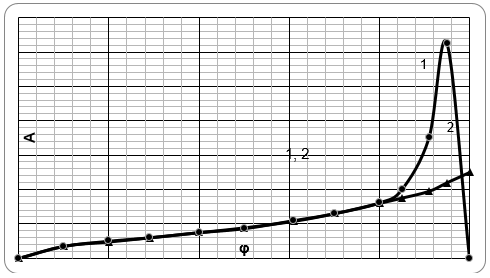Fig. 9. Isotherms of water vapors sorption by CMC (1) and ADC (2)

5.3. Sorption of water vapors by hydroxyethylcellulose (HEC)

Van Krevelen’s method of additive functions makes it possible to calculate sorption of water vapors by amorphous HEC with various degrees of substitution (DS). As follows from the calculations, introduction of hydroxyethyl radicals into cellulose doesn’t change content of hydroxyl groups and molar content of sorbed water molecules. However, an increase of DS and molecular weight of the HEC link leads to a decline in sorption values of the derivatives (A, g/g) expressed in grams of water per gram of HEC (Fig. 10).

The obtained sorption parameters of eq. (7) for calculation isotherms of HEC with various DS are shown in the Table 4. In particular, parameters for calculation isotherm of the commercial HEC sample (DS=2.4) were as follows:

A=0.33 g/g, g=0.87 kJ/mole and K=2.84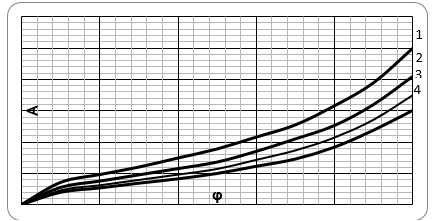Fig. 10. Isotherms of water vapors absorption by ADC (1) and amorphous HEC with DS=1 (2); DS=2 (3); and DS=3 (4)

5.4. Sorption of water vapors by methylcellulose (MC)

According to Van Krevelen’s method of additive functions, substitution of hydrogen atoms in hydroxyl groups of cellulose with non-polar methyl radicals should cause sharply decreasing hydrophility of this cellulose ether. After determination sorption parameters for MC having various DS (Table 4), isotherms of water vapor absorption can be calculated by eq. (7) (see Fig.11).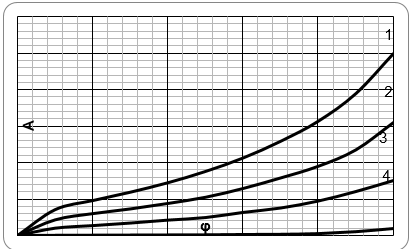Fig. 11. Isotherms of water vapors absorption by ADC (1) and amorphous MC with DS=1 (2); DS=2 (3); and DS=3 (4)

The calculated isotherms of water vapor absorption by commercial water-soluble cellulose derivatives, HEC and MC, coincide with experimental isotherms over a wide range of relative vapor pressures (Fig. 12). However at φ>0.8 the experimental isotherm greatly deviates from the calculated one, owing to the beginning of the unrestricted swelling (multimolecular water absorption) and forming a solution of the polymers in water.

If the sorption value A0.7 (g/g) at φ=0.7 is considered, then a linear correlation between A0.7 and DS of cellulose derivatives is observed (Fig. 13). This permits calculation of the degree of substitution of a derivative from sorption experiments using the Eq. (14) for HEC and Eq. (15) for MC.

DS = 6.75 – 27.0 A0.7 (14)

DS = 3.0 – 11.7 A0.7 (15)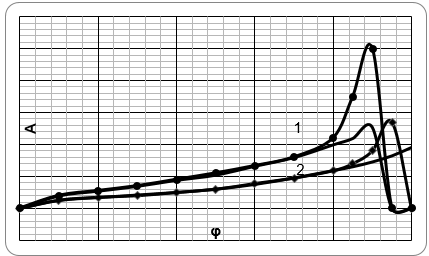Fig. 12. Isotherms of water vapors absorption by commercial HEC (1) and MC (2)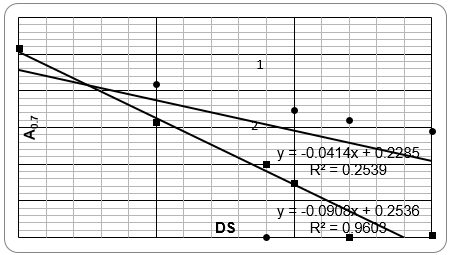Fig. 13. Correlation between A0.7 and DS for samples of HEC (1) and MC (2)

As can be seen from Table 6, the calculated DS-values are similar to the experimentally obtained values.

Table 6. Experimental (DSe) and Calculated (DSc) Degree of Substitution for some Cellulose Derivatives

###CONCLUSION

Sorption of vapors of various non-polar and polar liquids by celluloses and some cellulose derivatives was studied at 25 oC. The investigated samples had various degrees of crystallinity (from 0 to 0.75), content of amorphous domains (from 0.25 to 1), and specific surface area measured by nitrogen adsorption (from 0.4 to 110 m2/g). This specific surface was caused by nano-size mesopores.

Based on Gibbs-Duhem thermodynamics a simple equation was proposed, A=Ao/[1(RT/g)lnφ]to calculate the sorption isotherms. Depending on the g-value, this equation can describe isotherms of various shapes. If value of g is high (tens or hundreds kJ/mole), then an isotherm will have the saturation plateau; medium g-value (some unities of kJ/mole) gives a sigmoidal isotherm, while small g-value leads to an exponential isotherm. It was shown that calculated isotherms of monomolecular adsorption and absorption are close to experimentally obtained isotherms over a wide range of relative vapor pressures. In the case of sorption of water vapor by cellulose and its derivatives Van Krevelen’s method of additive functions can be used in order to predict contents of sorbed water by the polymer samples.

Application of the proposed equation makes it possible to calculate such structural characteristics of the polymers as accessible specific surface and crystallinity, as well as substitution degree of cellulose derivatives. Moreover, amounts of monomolecular and multimolecular fractions of the sorbate can be determined.

REFERENCES CITED

Allen, T. (1997). Particle Size Measurement: Surface Area and Pore Size Determination, Fifth Ed., Chapman & Hall, London.

Bariska, M., and Popper, R. (1975). “Ammonia sorption isotherms of wood and cotton cellulose,” Wood Sci. Technol. 9(2), 153-163.

Barnes, H. M., Skaar, C., and Luner P. (1977). “Water vapor sorption by amylose and cellulose acetates,” J. Appl. Polym. Sci. 21(4), 1085-1094.

Baumgartner, S., Kristl, J., and Peppas, N. A. (2002). “Network structure of cellulose ethers used in pharmaceutical applications during swelling and at equilibrium,” Pharmaceutic. Res.,19(8), 1084-1090.

Bikales, N., and Segal, L. (1971). Cellulose and Cellulose Derivatives, Wiley-Intersciences, New York.

Blair, T. C., Buckton, G., Beezer, A. E., and Bloomfield, S. F. (1990). “The interaction of various types of microcrystalline cellulose and starch with water,” Int. J. Pharmac. 63 (3), 251-257.

Brunauer, S, Emmett, P. H., and Teller, E. (1938). “Adsorption of gases in multimolecular layers,” J. Amer. Chem. Soc., 60, 309-319.

Chirkova, J., Andrsons, B., and Andersone, I. (2007). “Study of the structure of wood-related biopolymers by sorption methods,” BioResources 4(3), 1044-1057.

Dollimore, D., and Holt, B. (1973). “Sorption of vapors in cellulose film,” J. Appl. Polym. Sci., 17 (6), 1795-1803.

Gregg, S. I., and Sing, K. S. W. (1982). Adsorption, Surface Area and Porosity, Acad. Press, London, New York.

Gocho, H., Shimizu, H., Tanioka, A., Chou. T.-J., and Nakajima, T. (2000). “Effect of acetyl content on the sorption isotherm of water by cellulose acetate: Comparison with the thermal analysis results,” Carbohydrate Polymers, 41(1), 83-86.

Hermans, P. H. (1949). Physics and Chemistry of Cellulose Fibers, Elsevier Publ. Co., Amsterdam.

Hill, C. A. S., Norton, A. J., and Newman, G. (2009). “The water vapor sorption behavior of natural fibers,” J. Appl. Polym. Sci. 112, 1524-1537.

Ioelovich, M., and Kreitus, A. (1983). “Analytical description of hydrophilic properties of cellulose,” Wood Chemistry 3, 3-6.

Ioelovich, M. (1992). “Supermolecular structure of native and isolated cellulose,” Acta Polymerica 43, 110-113.

Ioelovich, M., and Gordeev, M. (1994). “Crystallinity of cellulose and its accessibility during deuteration,” Acta Polymerica 45, 121-123.

Ioelovich, M. (2009). “Accessibility and crystallinity of cellulose,” BioResources 4(3), 1168-1177.

Jeffries, R. (1960). “The sorption of water by cellulose and eight other textile materials,” J. Text. Inst. 51(3), 339-374.

Kawai, T. (1959). “Sorption of water vapor by cellulose and polymers at high humidities,” J. Polym. Sci. 37(131), 181-198.

Mandelkern, L., and Long, F. A. (1951). “Rate of sorption of organic vapors by films of cellulose acetate,” J. Polym. Sci. 6 (4), 457-469.

Mihranyan, A., Llagostera, A. P., Karmhag, R., Strømme, M., and Ek, R. (2004). “Moisture sorption by cellulose powders of varying crystallinity,” Int. J. Pharmac. 269 (2), 433-442.

Papkov, S. P., and Fainberg, E. Z. (1976). Interaction of Cellulose and Cellulosic Materials with Water, Chemistry, Moscow.

Rowen, J. W, and Blaine, R. L. (1947). “Sorption of nitrogen and water vapor on textile fibers,” Ind. Eng. Chem. 39(12), 1659-1663.

Sohn, S., and Kim, D. (2005). “Modiﬁcation of Langmuir isotherm in solution systems – Deﬁnition and utilization of concentration dependent factor,” Chemosphere 58, 115-123.

Tager, A. A. (1978). Physical Chemistry of Polymers, Chemistry, Moscow.

Urquhart, A. R. (1959). “Sorption of water on cellulose and starch,” In: Recent Advances in the Chemistry of Cellulose and Starch, Heywood and Co., London.

Van Krevelen, D. W. (1972). Properties of Polymers. Correlation with Chemical Structure, Elsevier Publ. Co., Amsterdam, London, New York.

Weatherwax, R. C., and Caulfield, D. E. (1971). “Cellulose aerogels: An improved method for preparing a highly expanded form of dry cellulose,” Tappi J. 54(6), 985-986.

Zografia, G., Kontnya, M. J., Yangb, A. Y. S., and. Brennerb, G. S. (1984). “Surface area and water vapor sorption of microcrystalline cellulose,” Int. J. Pharmac. 18 (1-2), 99-116.

Article submitted: October 18, 2010; Peer review completed: Nov. 20, 2010; Revised version received and accepted: Nov. 24, 2010; Published: Nov. 25, 2010.